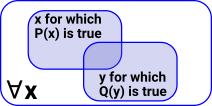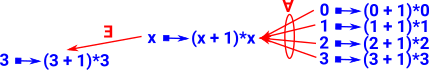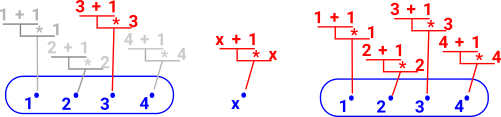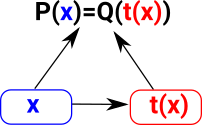# Maths - Category Theory - Substitution

 Imagine we have a property (a predicate) P that is true for all values of xWe can substitute y for x where y is a function of x:• The universal quantifier is the right adjoint of substitution.
• The existential quantifier is the left adjoint of substitution.

Πx:T -->
<-- (-)×T
Σx:T -->

As a sort of intuitive justification for this:

If we have a total function:

f: x -> y

Then we are saying 'y' exists for all 'x'.

https://ncatlab.org/nlab/show/substitution

### Substitute Variables and Literals

In type theory the deconstruct + construct (compute) allows us to substitute one variable for another.

Can we substitute between variables and literal values?If we start with a variable we can always go to a literal value () but all literal values must apply () before we can convert to a variable.

The following diagram is my attempt to draw this as fibre bundles:See Bartosz Milewski on his blog here.

 existential,sum Σweakening (adding an extra assumption) weakeninguniversal, product Π

### Substitute Variables and Expressions (Terms)

We have seen interesting structure when we substitute between variables and literals, now lets try substituting between variables and expressions.

### In Type Theory

Use 'context extension' instead of substitution.

https://ncatlab.org/nlab/show/context+extension

### In Category Theory

#### Pullback

 Substitution of a term into a predicate is pullback, but substitution of a term into a term is composition. Or if you are a type theorist: Substitution of a term into a dependent type is pullback, but substitution of a term into a term is composition. http://math.andrej.com/2012/09/28/substitution-is-pullback/Discussed more on these pages:

• More about binding variables and substitution on the type theory pages.
• More about substitution and lambda functions on the page here .

### Other Pages on this site

• Category of graphs described on page here.
• Implementing Graphs in FriCas program is discussed on page here.
• Implementing Posets in FriCas program is discussed on page here.

Where I can, I have put links to Amazon for books that are relevant to the subject, click on the appropriate country flag to get more details of the book or to buy it from them.Conceptual Mathematics - This is a book about category theory that does not assume an extensive knowledge over a wide area of mathematics. The style of the book is a bit quirky though.